The Wheatstone Bridge Circuit

The Wheatstone bridge can be used in various ways to measure electrical resistance:

• For the determination of the absolute value of a resistance by comparison with a known resistance
• For the determination of relative changes in resistance

The latter method is used with regard to strain gauge techniques. It enables relative changes of resistance in the strain gauge, which are usually around the order of 10-4 to 10-2 Ω/Ω to be measured with great accuracy.

The image below shows two different illustrations of the Wheatstone bridge which are electrically identical: figure a) shows the usual rhombus representation in which the Wheatstone is used; and figure b) is a representation of the same circuit, which will be clearer for an electrically untrained person.

The four arms or branches of the bridge circuit are formed by the resistances R1 to R4. The corner points 2 and 3 of the bridge designate the connections for the bridge excitation voltage Vs. The bridge output voltage V0 , that is the measurement signal, is available on the corner points 1 and 4.

Note: There is no generally accepted rule for the designation of the bridge components and connections. In existing literature, there are all kinds of designations and this is reflected in the bridge equations. Therefore, it is essential that the designations and indices used in the equations are considered along with their positions in the bridge networks in order to avoid misinterpretation.

The bridge excitation is usually an applied, stabilized direct, or alternating voltage Vs. If a supply voltage Vs is applied to the bridge supply points 2 and 3, then the supply voltage is divided up in the two halves of the bridge R1, R2 and R4, R3 as a ratio of the corresponding bridge resistances, i.e., each half of the bridge forms a voltage divider.

The bridge can be imbalanced, owing to the difference in the voltages from the electrical resistances on R1, R2 and R3, R4. This can be calculated as follows:

if the bridge is balanced and

where the bridge output voltage V0 is zero.

With a preset strain, the resistance of the strain gauge changes by the amount ΔR. This gives us the following equation:

For strain measurements, the resistances R1 and R2 must be equal in the Wheatstone bridge. The same applies to R3 and R4.

With a few assumptions and simplifications, the following equation can be determined (further explanations are given in the HBM book 'An Introduction to Measurements using Strain Gauges'):

In the last step of calculation, the term ΔR/R must be replaced by the following:

Here k is the k-factor of the strain gauge, ε is the strain. This gives us the following:

The equations assume that all the resistances in the bridge change. For instance, this situation occurs in transducers or with test objects performing similar functions. In experimental tests, this is hardly ever the case and usually only some of the bridge arms contain active strain gauges, the remainder consisting of bridge completion resistors. Designations for the various forms, such as quarter bridge, half bridge, double quarter or diagonal bridge and full bridge, are commonplace.

Depending on the measurement task one or more strain gauges are used at the measuring point. Although designations such as full bridge, half bridge, or quarter bridge are used to indicate such arrangements, actually they are not correct. In fact, the circuit used for the measurement is always complete and is either fully or partially formed by the strain gauges and the specimen. It is then completed by fixed resistors, which are incorporated within the instruments.

Transducers generally have to comply with more stringent accuracy requirements than measurements pertaining to experimental tests. Therefore, transducers should always have a full bridge circuit with active strain gauges in all four arms.

Full bridge or half bridge circuits should also be used for stress analysis if different kinds of interferences need to be eliminated. An important condition is that cases of different stresses are clearly distinguished, such as compressive or tensile stress, as well as bending, shear, or torsional forces.

The table below shows the dependence of the geometrical position of the strain gauges, the type of bridge circuit used and the resulting bridge factor B for normal forces, bending moments, torque and temperatures. The small tables given for each example specify the bridge factor B for each type of influencing quantity. The equations are used to calculate the effective strain from the bridge output signal VO/VS.

 Bridge configuration External impacts measured: Application Description Advantages and disadvantages 1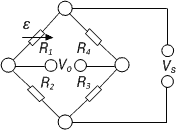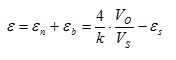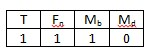Strain measurement on a tension/ compression bar Strain measurement on a bending beam Simple quarter bridge Simple quarter bridge circuit with one active strain gauge + Easy installation - Normal and bending strain are superimposed - Temperature effects not automatically compensated 2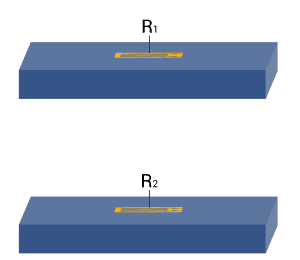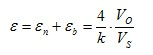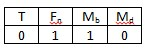Strain measurement on a tension/ compression bar Strain measurement on a bending beam Quarter bridge with an external dummy strain gauge Two quarter bridge circuits, one actively measures strain, the other is mounted on a passive component made of the same material, which is not strained + Temperature effects are well compensated - Normal and bending strain cannot be separated (superimposed bending) 3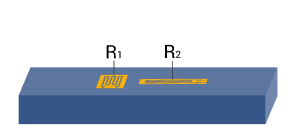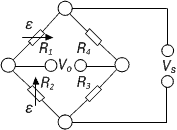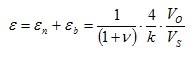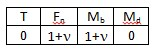Strain measurement on a tension/ compression bar Strain measurement on a bending beam Poisson half-bridge Two active strain gauges connected as a half bridge, one of them positioned at 90° to the other + Temperature effects are well compensated when material is isotrop 4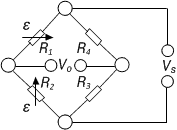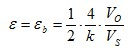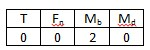Strain measurement on a bending beam Half bridge Two strain gauges are installed on opposite sides of the structure + Temperature effects are well compensated + Separation of normal and bending strain (only the bending effect is measured) 5Strain measurement on a tension/ compression bar Diagonal bridge Two strain gauges are installed on opposite sides of the structure + Normal strain is measured independently of bending strain (bending is excluded) 6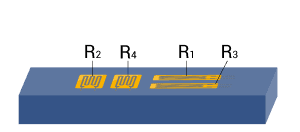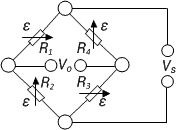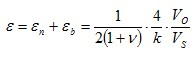Strain measurement  on a tension/ compression bar Strain measurement on a bending beam Full bridge 4 strain gauges are installed on one side of the structure as a full bridge + Temperature effects are well compensated + High output signal and excellent common mode rejection (CMR) - Normal and bending strain cannot be separated (superimposed bending) 7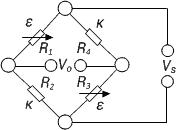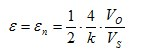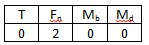Strain measurement on a tension/ compression bar Diagonal bridge with dummy gauges Two active strain gauges, two passive strain gauges + Normal strain is measured independently of bending strain (bending is excluded) + Temperature effects are well compensated 8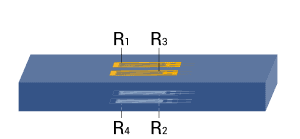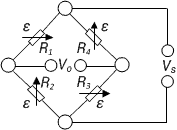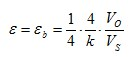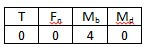Strain measurement on a bending beam Full bridge Four active strain gauges are connected as a full bridge + Separation of normal and bending strain (only the bending effect is measured) + High output signal and excellent common mode rejection (CMR) +Temperature effects are well compensated 9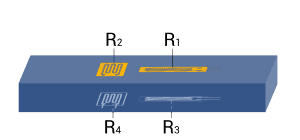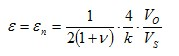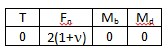Strain measurement on a tension/ compression bar Full bridge Four active strain gauges, two of them rotated by 90° + Normal strain is measured independently of bending strain (bending is excluded) + Temperature effects are well compensated + High output signal and excellent common mode rejection (CMR) 10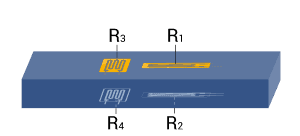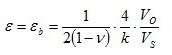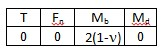Strain measurement on a bending beam Full bridge Four active strain gauges, two of them rotated by 90° + Separation of normal and bending strain (only the bending effect is measured) + Excellent common mode rejection (CMR) + Temperature effects are well compensated 11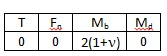Strain measurement on a bending beam Full bridge Four active strain gauges, two of them rotated by 90° + Separation of normal and bending strain (only the bending effect is measured) + High output signal and excellent common mode rejection (CMR) + Temperature effects are well compensated 12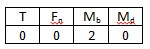Strain measurement on a bending beam Half bridge Four active strain gauges connected as a half bridge + Separation of normal and bending strain (only the bending effect is measured) + Temperature effects are well compensated + High output signal and excellent common mode rejection (CMR) 13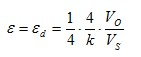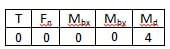Measurement of torsion strain Full bridge Four strain gauges are installed, each at an angle of 45° to the main axis as shown + High output signal and excellent common mode rejection (CMR) + Temperature effects are well compensated 14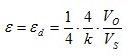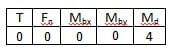Measurement of torsion strain with limited space for installation Full bridge Four strain gauges are installed as a full bridge, at an angle of 45° and superimposed (stacked rosettes) + High output signal and excellent common mode rejection (CMR) + Temperature effects are well compensated 15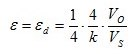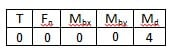Measurement of torsion strain with limited space for installation Full bridge Four strain gauges are installed as a full bridge at an angle of 45° and superimposed (stacked rosettes) + High output signal and excellent common mode rejection (CMR) + Temperature effects are well compensated

Note: A cylindrical shaft is assumed for torque measurement in example 13, 14, and 15. For reasons related to symmetry, bending in X and Y direction is allowed. The same conditions also apply for the bar with square or rectangular cross sections.

Explanations of the symbols:

 T Temperature Fn Normal force Mb Bending moment Mbx, Mby Bending moment for X and Y directions Md Torque εs Apparent strain εn Normal strain εb Bending strain εd Torsion strain ε Effective strain at the point of measurement ν Poisson’s ratio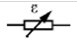Active strain gauge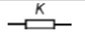Strain gauge for temperature compensation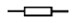Resistor or passive strain gauge